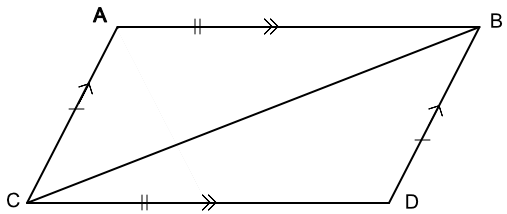Geometric Proofs

# Geometric Proofs

GCSE(H),

Geometric Proofs use a logical argument that always follows the same form.

Proofs start with a Given fact about the problem being solved. There then follows one or more steps, which consist of a Statement followed by the Reason that that statement is true.

The final statement is the fact being proven.

See Congruent Triangles for reasons that can be used in these proofs.

## Examples

1. For the parallelogram given below, prove that angle CAB is congruent to angle BDC. You may assume that opposite sides in a parallelogram are equal.Answer: Given: Parallelogram ABCD with a diagonal BC;

Opposite sides in a parallelogram are equal

StatementReason
AB = CDOpposite sides in a parallelogram are equal
AC = BDOpposite sides in a parallelogram are equal
BC is sharedLine BC shared by both triangles
Triangles are congruentCongruency by SSS; therefore ∠CAB = ∠BDC

2. Prove that, for the triangle below, a line drawn at a right angle from the midpoint of AB (point D) bisects the angle at C.Answer: Given: The triangle is isosceles; D is the midpoint of AB

StatementReason
DC is commonLine shared by both smaller triangles
AC = CBDefinition of an isosceles triangle
AD = DBD is given as the midpoint
Triangles are congruentCongruency by SSS
∠ACD = ∠DCB∠ACB is bisected